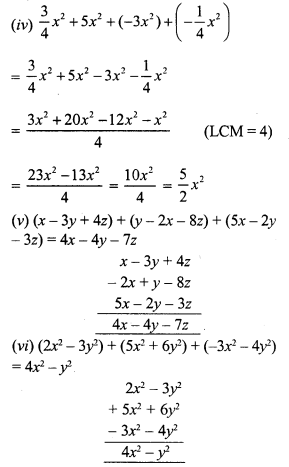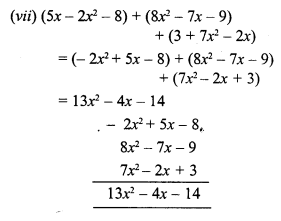# RS Aggarwal Class 7 Solutions Chapter 6 Algebraic Expressions Ex 6A

In this chapter, we provide RS Aggarwal Solutions for Class 7 Chapter 6 Algebraic Expressions Ex 6A for English medium students, Which will very helpful for every student in their exams. Students can download the latest RS Aggarwal Solutions for Class 7 Chapter 6 Algebraic Expressions Ex 6A Maths pdf, free RS Aggarwal Solutions Class 7 Chapter 6 Algebraic Expressions Ex 6A Maths book pdf download. Now you will get step by step solution to each question.

### RS Aggarwal Solutions for Class 7 Chapter 6 Exponents Ex 6A Download PDF

Question 1.
Solution:Question 2.
Solution:Question 3.
Solution:
Sum of (a + 3b – 4c), (4a – b + 9c) and (-2b + 3c – a)Now subtract (2a – 3b + 4c) from 4a + 8c
= 4a + 8c – (2a – 3b + 4c)
= 4a + 8c – 2a + 3b – 4c
= 4a – 2a + 3b + 8c – 4c
= 2a + 3b + 4c

Question 4.
Solution:Question 5.
Solution:
Sum of (8a – 6a² + 9) and (-10a – 8 + 8a²)
= 8a – 6a² + 9 + (-10a) – 8 + 8a²
= 8a – 10a – 6a² + 8a² + 9 – 8
= -2a + 2a² + 1
Now -3 – (-2a + 2a² + 1)
= (-3 + 2a – 2a² – 1)
= -4 + 2a – 2a²

Question 6.
Solution:All Chapter RS Aggarwal Solutions For Class 7 Maths

—————————————————————————–

All Subject NCERT Exemplar Problems Solutions For Class 7

All Subject NCERT Solutions For Class 7

*************************************************

I think you got complete solutions for this chapter. If You have any queries regarding this chapter, please comment on the below section our subject teacher will answer you. We tried our best to give complete solutions so you got good marks in your exam.

If these solutions have helped you, you can also share rsaggarwalsolutions.in to your friends.